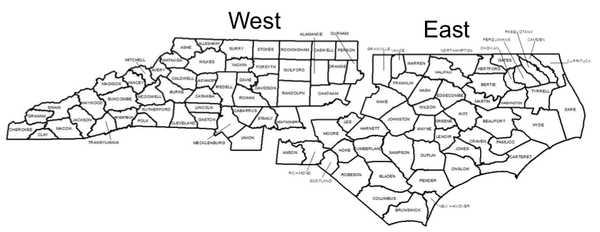NC State Extension Publications

## Introduction

Loggers, timber buyers, and others have good reasons to be interested in historic North Carolina delivered timber prices, prices paid upon delivery of the timber to the mill. Not only is such information useful in assessing current market opportunities, but may also be useful in analyzing business trends. Delivered prices provides insight into the compensation loggers may receive for all their costs, plus a minor profit for their labors. Delivered prices consist of the price paid to landowners for their standing timber + cutting + skidding + loading + hauling + other fixed costs + variable costs.

These historic price data have been compiled from various sources (primarily Timber Mart-South since 1988). Annual market prices are averages of delivered prices reported at the time. Considerable additional information on these products and others, as well as other states and regions, are available from the collectors and distributors of price information.

Delivered price data are reported for four timber product categories (pine sawtimber and pulpwood, and mixed hardwood sawtimber and pulpwood) for western North Carolina, eastern North Carolina, statewide, and southeast, United States. Eastern North Carolina is defined as the region east of, and including, Granville, Wake, Lee, Moore, Richmond and Anson counties (Figure 1). Western North Carolina is defined as the region west of and including Person, Durham, Chatham, Montgomery, Stanley, and Union counties (Figure 1).Figure 1: Map of North Carolina counties divided into two regions, east and west.

Created by Jessica Knight

## Pine Sawtimber Delivered Price History ($/1000 board feet, Scribner log rule) Skip to Pine Sawtimber Delivered Price History ($/1000 board feet, Scribner log rule)
 Year Eastern NC Western NC State-wide Average Southeast-wide Average 1988 $206.00$164.00 $188.00 1989$224.00 $162.00$194.00 1990 $240.00$160.00 $199.00 1991$230.00 $166.00$192.00 1992 $232.00$173.00 $203.00$222.00 1993 $264.00$187.00 $226.00$256.00 1994 $287.00$231.00 $259.00$316.00 1995 $295.00$233.00 $264.00$349.00 1996 $355.00$200.00 $277.00$329.00 1997 $404.00$205.00 $304.00$379.00 1998 $423.00$235.00 $329.00$402.00 1999 $384.00$243.00 $314.00$373.00 2000 $429.00$282.00 $356.00$388.00 2001 $390.00$269.00 $330.00$354.00 2002 $400.00$294.00 $347.00$361.00 2003 $397.00$346.00 $371.00$366.00 2004 $371.00$277.00 $324.00$381.00 2005 $435.00$312.00 $374.00$406.00 2006 $406.00$315.00 $360.00$395.00 2007 $399.00$307.00 $353.00$380.00 2008 $369.00$318.00 $343.00$360.00 2009 $341.00$288.00 $314.00$318.00 2010 $371.00$305.00 $338.00$333.00 2011 $338.00$275.00 $306.00$311.00 2012 $319.00$302.00 $310.00$310.00 2013 $347.00$319.00 $333.00$323.00 2014 $346.00$310.00 $328.00$334.00 2015 $338.00$293.00 $315.00$335.00 2016 $361.00$292.75 $327.25$328.75 2017 $342.50$287.50 $315.00$320.75 2018 $342.65$290.28 $316.54$319.25 2019 $385.75$284.25 $353.23$328.50 2020 $370.50$259.50 $315.25$305.75 2021 $423.46$330.98 $377.44$338.87 2022 $390.05$316.75 $353.40$39.00

## Pine Pulpwood Delivered Price History ($/Cord, Scribner log rule) Skip to Pine Pulpwood Delivered Price History ($/Cord, Scribner log rule)
 Year Eastern NC Western NC State-wide Average Southeast-wide Average 1988 $42.18$42.31 $42.68 1989$44.00 $46.00$45.46 1990 $46.39$49.14 $48.55 1991$48.50 $49.18$49.06 1992 $48.58$45.26 $46.92$51.89 1993 $38.60$53.30 $45.95$56.65 1994 $54.03$50.37 $52.20$56.53 1995 $61.00$51.00 $56.00$61.70 1996 $49.78$48.14 $48.96$59.88 1997 $54.63$49.17 $51.90$65.62 1998 $58.39$48.85 $53.62$67.32 1999 $48.33$48.37 $48.35$61.38 2000 $56.14$49.47 $52.81$58.22 2001 $56.34$44.16 $50.25$56.20 2002 $56.24$46.55 $51.39$56.96 2003 $54.52$51.16 $52.84$60.46 2004 $57.96$49.89 $53.93$60.31 2005 $57.57$55.57 $56.57$66.77 2006 $59.99$56.83 $58.41$64.16 2007 $61.43$60.18 $60.81$68.11 2008 $64.10$62.94 $63.52$73.27 2009 $68.89$64.56 $66.72$68.77 2010 $70.02$63.97 $66.99$73.63 2011 $68.27$61.34 $64.81$70.03 2012 $69.14$64.59 $66.87$73.07 2013 $72.39$66.34 $69.36$76.21 2014 $82.22$67.86 $75.04$80.12 2015 $75.84$64.47 $70.15$79.73 2016 $83.28$68.53 $75.91$79.91 2017 $92.71$72.80 $82.75$78.41 2018 $91.86$72.46 $82.17$82.23 2019 $86.46$71.85 $79.20$78.77 2020 $81.99$67.72 $74.86$75.50 2021 $90.94$74.50 $82.73$80.72 2022 $91.47$82.99 $87.23$87.09

## Mixed Hardwood Sawtimber Delivered Price History ($/1000 board feet, Doyle log rule) Skip to Mixed Hardwood Sawtimber Delivered Price History ($/1000 board feet, Doyle log rule)
 Year Eastern NC Western NC State-wide Average Southeast-wide Average 1988 $148.00$165.00 $160.00 1989$157.00 $166.00$162.00 1990 $181.00$163.00 $173.00 1991$188.00 $154.00$173.00 1992 $211.00$192.00 $201.00$176.00 1993 $203.00$205.00 $204.00$226.00 1994 $246.00$202.00 $224.00$217.00 1995 $229.00$211.00 $220.00$243.00 1996 $265.00$237.00 $251.00$238.00 1997 $264.00$261.00 $262.00$262.00 1998 $245.00$261.00 $253.00$273.00 1999 $194.00$259.00 $226.00$257.00 2000 $251.00$249.00 $249.00$267.00 2001 $279.00$250.00 $265.00$282.00 2002 $286.00$302.00 $294.00$292.00 2003 $236.00$279.00 $257.00$316.00 2004 $253.00$263.00 $258.00$318.00 2005 $289.00$303.00 $297.00$332.00 2006 $288.00$290.00 $289.00$322.00 2007 $305.00$294.00 $299.00$332.00 2008 $302.00$334.00 $318.00$346.00 2009 $297.00$290.00 $293.00$336.00 2010 $336.00$307.00 $322.00$355.00 2011 $350.00$316.00 $333.00$355.00 2012 $359.00$331.00 $345.00$373.00 2013 $394.00$364.00 $379.00$400.00 2014 $431.00$411.00 $421.00$445.00 2015 $431.00$356.00 $393.00$458.00 2016 $414.75$325.75 $370.25$466.50 2017 $398.50$364.00 $381.25$455.00 2018 $393.95$367.68 $382.89$454.04 2019 $452.50$428.50 $432.11$468.33 2020 $433.00$410.00 $421.75$468.75 2021 $457.72$421.40 $439.65$463.68 2022 $574.50$526.00 $550.25$521.25

## Hardwood Pulpwood Delivered Price History ($/Cord Doyle log rule) Skip to Hardwood Pulpwood Delivered Price History ($/Cord Doyle log rule)
 Year Eastern NC Western NC State-wide Average Southeast-wide Average 1988 $34.71$37.15 $37.12 1989$38.38 $44.00$41.67 1990 $42.50$47.05 $45.06 1991$40.88 $46.43$44.26 1992 $46.50$44.07 $45.29$43.92 1993 $49.49$48.10 $48.79$50.01 1994 $51.78$51.60 $51.69$50.33 1995 $55.00$50.00 $52.50$57.25 1996 $49.06$52.96 $51.00$54.50 1997 $54.90$53.49 $54.19$62.01 1998 $56.03$52.73 $54.38$60.54 1999 $49.19$54.19 $51.69$54.43 2000 $60.08$55.94 $58.01$54.88 2001 $57.90$52.54 $55.21$60.94 2002 $53.75$53.70 $53.72$61.21 2003 $54.56$66.26 $60.41$68.86 2004 $56.74$61.37 $59.06$65.06 2005 $61.21$63.68 $62.45$72.80 2006 $61.29$63.68 $62.49$67.05 2007 $62.64$66.70 $64.67$69.51 2008 $70.57$73.85 $72.21$78.18 2009 $68.21$73.28 $70.75$76.25 2010 $69.09$72.31 $70.70$82.52 2011 $68.59$70.26 $69.43$74.41 2012 $68.07$66.92 $67.49$78.19 2013 $67.48$67.66 $67.57$83.71 2014 $76.46$72.58 $74.52$91.00 2015 $74.40$75.22 $74.81$89.00 2016 $71.86$73.68 $72.77$87.36 2017 $67.80$71.36 $69.58$83.49 2018 $65.14$74.70 $69.91$88.90 2019 $75.90$82.86 $79.36$94.23 2020 $74.62$75.84 $75.23$85.36 2021 $85.80$81.51 $83.65$95.77 2022 $84.20$91.65 $87.93$105.52

# Author

Associate Dean of Extension, Department Extension Leader & Professor
Forestry & Environmental Resources